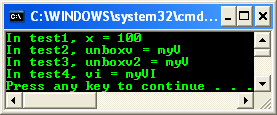4. # safe_cast and Unboxing

safe_cast and Generic Types

The following sample shows that safe_cast can perform a downcast with a generic type.

// mysafecast.cpp : main project file - safe_cast and generic types.

// compile with: /clr

#include "stdafx.h"

using namespace System;

interface struct myI {/* more code */};

generic<class myT> where myT:myI

ref struct Base

{

myT t;

void test1() {/* more code */}

};

generic<class myT> where myT:myI

ref struct Derived:public Base <myT> {/* more code */};

ref struct myR:public myI {/* more code */};

typedef Base<myR^> GBase_myR;

typedef Derived<myR^> GDerived_myR;

int main(array<System::String ^> ^args)

{

GBase_myR^ br = gcnew GDerived_myR();

GDerived_myR^ dr = safe_cast<GDerived_myR^>(br);

return 0;

}

// no output

safe_cast and User-Defined Conversions (UDC)

The following sample shows that user-defined conversions can be invoked by a safe_cast.

// mysafecast.cpp : main project file - safe_cast and

// user defined conversion, UDC

// compile with: /clr

#include "stdafx.h"

using namespace System;

value struct myV;

ref struct myR

{

int x;

myR() { x = 1; }

myR(int argx) { x = argx; }

static operator myR::myV^(myR^ r);

};

value struct myV

{

int x;

static operator myR^(myV& v)

{

Console::WriteLine("In operator myR^(myV& v)");

myR^ r = gcnew myR();

r->x = v.x;

return r;

}

myV(int argx) { x = argx; }

};

myR::operator myV^(myR^ r)

{

Console::WriteLine("In operator myV^(myR^ r)");

return gcnew myV(r->x);

}

int main(array<System::String ^> ^args)

{

bool fReturnVal = false;

myV v(2);

// should invoke UDC

myR^ r = safe_cast<myR^>(v);

// should invoke UDC

myV^ v2 = safe_cast<myV^>(r);

return 0;

}

Output: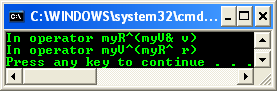safe_cast and Boxing

Boxing in defined as a compiler-injected, user-defined conversion. Therefore, safe_cast can be used to box a value on the CLR heap. The following sample shows boxing with simple and user defined value types. A safe_cast will box a value type variable that is on the native stack so it can be assigned to a variable on the garbage-collected heap. Try the following example by using the previous project.

// mysafecast.cpp : main project file - safe_cast and boxing.

// compile with: /clr

#include "stdafx.h"

using namespace System;

interface struct myI {/* more code */};

value struct myV : public myI

{

int m_x;

myV(int i) : m_x(i) {/* more code */}

};

int main(array<System::String ^> ^args)

{

// box a value type

myV v(100);

// myV to myI

myI^ i = safe_cast<myI^>(v);

Console::WriteLine("i = {0}", i);

int x = 200;

myV^ refv = safe_cast<myV^>(v);

Console::WriteLine("x = {0}", x);

int^ refi = safe_cast<int^>(x);

Console::WriteLine("refi = {0}", refi);

return 0;

}

Output: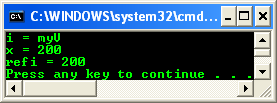The following sample shows that boxing has a higher priority over a user-defined conversion in a safe_cast operation:

// mysafecast.cpp : main project file – another safe_cast and boxing

// compile with: /clr

#include "stdafx.h"

using namespace System;

static bool fRetval = true;

interface struct myI {/* more code */};

value struct myV : public myI

{

int x;

myV(int argx)

{ x = argx; }

static operator myI^(myV v)

{

fRetval = false;

myI^ pi = v;

return pi;

}

};

ref struct myR

{

myR() {/* more code */}

myR(myV^ pv) {/* more code */}

};

int main(array<System::String ^> ^args)

{

myV v(100);

// boxing will occur, not UDC "operator I^"

myI^ pv = safe_cast<myI^>(v);

Console::WriteLine("pv = {0}", pv);

return 0;

}

Output:

---------------------------------------------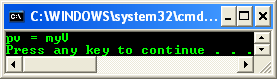safe_cast and Unboxing

Unboxing in defined as a compiler-injected, user-defined conversion. Therefore, safe_cast can be used to unbox a value on the CLR heap. Unboxing is a user-defined conversion, but unlike boxing, unboxing must be explicitly, which means it must be performed by a , C-style cast, or safe_cast; unboxing cannot be performed implicitly. For example:

// mysafecast.cpp : main project file - safe_cast unboxing

// compile with: /clr

#include "stdafx.h"

using namespace System;

int main(array<System::String ^> ^args)

{

System::Object ^ obj = 100;

// object to int

int x = safe_cast<int>(obj);

Console::WriteLine("x = {0}", x);

return 0;

}

Output: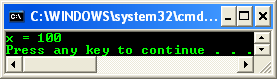The following sample shows unboxing with value types and primitive types.

// mysafecast.cpp : main project file - safe_cast and unboxing

// compile with: /clr

#include "stdafx.h"

using namespace System;

interface struct myI {};

value struct myVI : public myI {/* more code */};

void test1() {

Object^ obj = 100;

int x = safe_cast<Int32>(obj);

Console::WriteLine("In test1, x = {0}", x);

}

value struct myV {

int x;

String^ sobj;

};

void test2() {

myV localv;

Object^ obj = localv;

myV unboxv = safe_cast<myV>(obj);

Console::WriteLine("In test2, unboxv = {0}", unboxv);

}

void test3() {

myV localv;

myV^ obj2 = localv;

myV unboxv2 = safe_cast<myV>(obj2);

Console::WriteLine("In test3, unboxv2 = {0}", unboxv2);

}

void test4() {

myI^ refi = myVI();

myVI vi  = safe_cast<myVI>(refi);

Console::WriteLine("In test4, vi = {0}", vi);

}

int main(array<System::String ^> ^args)

{

test1();

test2();

test3();

test4();

return 0;

}

Output: